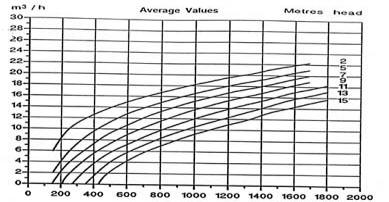The output power is the power applied to the fluid to meet the requirements of network throughput. The output power can be calculated as a function of flow and pressure:

output power Watt = flow rate (m3/sec) x Pressure (pascals)

The energies of pressure being in 3 forms (see Bernoulli), In a complete study we add the three energies pressure: head loss + hydrostatic load + dynamic pressure: output power ( Watt) = flow rate (m3/sec) x (head loss+ hydrostatic load + dynamic pressure)(pascals)

Example Output power with head loss: The output power of a fluid flow through a 2 m3/sec element generating a loss of 100 Pascals is 2x100 = 200

Example Output power with hydrostatic head pressure: calculating the power output of a water flow of 0.1 m3/sec from an altitude of 0 to 10 meters: start by turning the height of hydrostatic pressure in pascals: assume for simplicity that 1 bar = 100 000 pascals = 10 meters column water (see hydrostatic head) This gives us an output power: 0.1 x 100 000 = 10 000 watts

Example Output power with dynamic pressure: A pump delivers a flow rate of 1 m3/sec by tapping into a lake, and rejected the water at the same level through an output section of pipe 0.1m ².(pressure losses are neglected) This system generates a dynamic pressure as it brings the speed to the fluid, which was at rest

• Velocity given to the fluid is: flow (m3/sec) / section (m²)= 1/0.1=0.1m/sec
• The dynamic pressure energy is equal to 0.5 x density(kg/m3) x velocity(m/sec)²= 0.5 x 1000 x 0.1²=5 pascals
• Output power Watt = flow (m3/sec) x dynamic Pressure (pascals) =1x5=5 watts

In a complete study we add the three energies pressure: output power Watt = flow (m3/sec) x (head loss + hydrostatic head (pascals) + dynamic Pressure )

The ratio of the useful power by power consumption gives the efficiency of the pump.

• if the power consumption is measured at the shaft of the pump, the ratio of the output power by the power consumption gives the performance of the pump body without the engines
• If the power consumption is measured by power consumption of the pump motor, the ratio of effective power by the power input gives you the efficiency of your pump including engine efficiency.

This curve indicates for a given pump, the flow rates achieved according to power consumption..It can be seen for example with a power of 175W about this pump delivers: 6 M3 /h with 2 meters HMT, 2m3 with 5 mètres...